### Atoms And Molecules Class 10th Science Tamilnadu Board Solution

##### Question 1.When ammonia reacts with hydrogen chloride gas, it produces white fumes of ammonium chloride. The volume occupied by NH3 in glass bulb A is three times more than the volume occupied by HCl in glass bulb B at STP.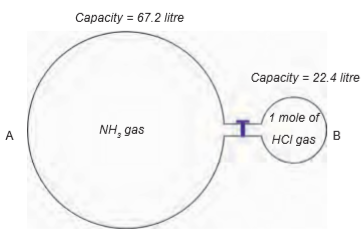i) How many moles of ammonia are present in glass bulb A?ii) How many grams of NH4Cl will be formed when the stopper is opened?(Atomic mass of N = 14, H = 1, Cl = 35.5)iii) Which gas will remain after completion of the reaction?iv) Write the chemical reaction involved in this process.Answer:(i) 3 moles(ii) 53.5 grams (14 + 4 + 35.5)(iii) Ammonia ( 2 moles will be remaining )(iv) NH3 + HCl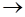NH4ClExplanation -1 mole of any gas at STP occupies 22.4 litres of volume. So, 1 mole of ammonia occupies 22.4 litres of volume. 67.2 litres (22.4 x 3) of volume is 3 moles of ammonia. 22.4 litres of HCl is 1 mole of HCl. According to reaction ,1 mole of ammonia reacts with 1 mole of HCl to form 1 mole of NH4Cl, which comes out as white fumes.NH3 + HClNH4ClEven though 3 moles of ammonia is present in bulb, only one 1 mole undergoes reaction with 1 mole of HCl. The other 2 moles of ammonia remain unreacted.Question 2.Nitro glycerine is used as an explosive. The equation for the explosive reaction is4C3H5((NO3))312CO2 + 10H2O + 6N2 + O2(l) (g) (l) (g) (g)(Atomic mass of C = 12, H = 1, N = 14, O=16)1. How many moles does the equation show for i) Nitroglycerine ii) gas molecules produced?2. How many moles of gas molecules are obtained from 1 mole of nitroglycerine?3. What is the mass of 1 mole of nitroglycerine?Answer:1. 4 moles of nitroglycerine ; CO2 (12) + N2(6) + O2(1) = 19 moles ofgas molecules are formed.2. 4 moles of nitroglycerine gives 19 moles of gas molecules. So, 1 moleof nitroglycerine gives (19/4) 4.75 moles of gas molecules.3. Gram molecular mass of nitroglycerine is 1 mole of nitroglycerine.So, mass of 1 mole of nitroglycerine is 227 grams.[(3x12)C + (5x1)H + (3x14)N + (9x16)O]Question 3.Sodium bi carbonate breaks down on heating:2NaHCO3Na2CO3 + H2O + CO2(Atomic mass of Na = 23, C = 12, H = 1, O=16)i) How many moles of sodium bi carbonate are there in the equation?ii) What is the mass of sodium bicarbonate used in this equation?iii) How many moles of carbon dioxide are there in this equation?Answer:(i) Two (coefficient of sodium bi carbonate)(ii) 1 mole of sodium bi carbonate contains 84 grams. Two moles ofsodium bi carbonate contains 168 grams. So, 168 grams of sodium bi carbonate is used in the equation.(iii) 1 mole (coefficient of CO2 )Question 4.40 g of calcium was extracted from 56 g of calcium oxide(Atomic mass of Ca= 40, O=16)i) What mass of oxygen is there in 56 g of calcium oxide?ii) How many moles of oxygen atoms are there in this?iii) How many moles of calcium atoms are there in 40 g of calcium?iv) What mass of calcium will be obtained from 1000 g of calcium oxide?Answer:(i) 16 grams of O(ii) 1 mole of oxygen atoms(iii) 1 mole(iv) 714.38 grams.56 grams of CaO gives 40 grams of Ca. 1000 grams of CaO gives (1000x40)/56 = 714.28 grams of calciumExplanation –CaO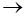Ca + O56 40 161 mole of any compound (or gas in molecular form) contains the mass equal to its gram molecular mass. 1 mole of any element contains the mass equal to its gram atomic mass. So,1 mole of CaO = 40 + 16 = 56 grams1 mole of Ca = 40 grams1 mole of oxygen atoms = 16 grams1 mole of calcium oxide dissociates to form 1 mole of Ca and 1 mole of O atoms.Question 5.How many grams are there in the following?i) 1 mole of chlorine molecule, Cl2ii) 2 moles of sulphur molecules, S8iii) 4 moles of ozone molecules, O3iv) 2 moles of nitrogen molecules, N2Answer:we know that when gases are present in the molecular form, 1 mole of any gas is equal to 6.023 x 1023 molecules of that gas, which is equal to the gram molecular weight of gas in the combined state.(i) 1 mole of chlorine molecules contains 71 grams of Cl2. (2×35.5)(ii) 2 moles of sulphur molecules contain 512 grams of S8. (2×32×8)(iii) 4 moles of ozone molecules contain 192 grams of O3. (4× 16× 3)(iv) 2 moles of nitrogen molecules contain 56 grams of N2. (2× 14× 2)Question 6.Find how many moles of atoms are there in:i) 2 g of nitrogen.ii) 23 g of sodiumiii) 40 g of calcium.iv) 1.4 g of lithiumv) 32 g of sulphur.Answer: We know the formula that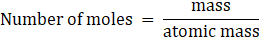Applying the above formula for all cases.(i)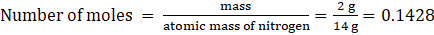(ii)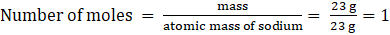(iii)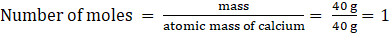(iv)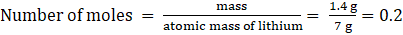(v)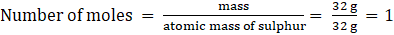PDF FILE TO YOUR EMAIL IMMEDIATELY PURCHASE NOTES & PAPER SOLUTION. @ Rs. 50/- each (GST extra)

HINDI ENTIRE PAPER SOLUTION

MARATHI PAPER SOLUTION

SSC MATHS I PAPER SOLUTION

SSC MATHS II PAPER SOLUTION

SSC SCIENCE I PAPER SOLUTION

SSC SCIENCE II PAPER SOLUTION

SSC ENGLISH PAPER SOLUTION

SSC & HSC ENGLISH WRITING SKILL

HSC ACCOUNTS NOTES

HSC OCM NOTES

HSC ECONOMICS NOTES

HSC SECRETARIAL PRACTICE NOTES

2019 Board Paper Solution

HSC ENGLISH SET A 2019 21st February, 2019

HSC ENGLISH SET B 2019 21st February, 2019

HSC ENGLISH SET C 2019 21st February, 2019

HSC ENGLISH SET D 2019 21st February, 2019

SECRETARIAL PRACTICE (S.P) 2019 25th February, 2019

HSC XII PHYSICS 2019 25th February, 2019

CHEMISTRY XII HSC SOLUTION 27th, February, 2019

OCM PAPER SOLUTION 2019 27th, February, 2019

HSC MATHS PAPER SOLUTION COMMERCE, 2nd March, 2019

HSC MATHS PAPER SOLUTION SCIENCE 2nd, March, 2019

SSC ENGLISH STD 10 5TH MARCH, 2019.

HSC XII ACCOUNTS 2019 6th March, 2019

HSC XII BIOLOGY 2019 6TH March, 2019

HSC XII ECONOMICS 9Th March 2019

SSC Maths I March 2019 Solution 10th Standard11th, March, 2019

SSC MATHS II MARCH 2019 SOLUTION 10TH STD.13th March, 2019

SSC SCIENCE I MARCH 2019 SOLUTION 10TH STD. 15th March, 2019.

SSC SCIENCE II MARCH 2019 SOLUTION 10TH STD. 18th March, 2019.

SSC SOCIAL SCIENCE I MARCH 2019 SOLUTION20th March, 2019

SSC SOCIAL SCIENCE II MARCH 2019 SOLUTION, 22nd March, 2019

XII CBSE - BOARD - MARCH - 2019 ENGLISH - QP + SOLUTIONS, 2nd March, 2019

HSC Maharashtra Board Papers 2020

(Std 12th English Medium)

HSC ECONOMICS MARCH 2020

HSC OCM MARCH 2020

HSC ACCOUNTS MARCH 2020

HSC S.P. MARCH 2020

HSC ENGLISH MARCH 2020

HSC HINDI MARCH 2020

HSC MARATHI MARCH 2020

HSC MATHS MARCH 2020

SSC Maharashtra Board Papers 2020

(Std 10th English Medium)

English MARCH 2020

HindI MARCH 2020

Hindi (Composite) MARCH 2020

Marathi MARCH 2020

Mathematics (Paper 1) MARCH 2020

Mathematics (Paper 2) MARCH 2020

Sanskrit MARCH 2020

Sanskrit (Composite) MARCH 2020

Science (Paper 1) MARCH 2020

Science (Paper 2)

MUST REMEMBER THINGS on the day of Exam

Are you prepared? for English Grammar in Board Exam.

Paper Presentation In Board Exam

How to Score Good Marks in SSC Board Exams

Tips To Score More Than 90% Marks In 12th Board Exam

How to write English exams?

How to prepare for board exam when less time is left

How to memorise what you learn for board exam

No. 1 Simple Hack, you can try out, in preparing for Board Exam

How to Study for CBSE Class 10 Board Exams Subject Wise Tips?

JEE Main 2020 Registration Process – Exam Pattern & Important Dates

NEET UG 2020 Registration Process Exam Pattern & Important Dates

How can One Prepare for two Competitive Exams at the same time?

8 Proven Tips to Handle Anxiety before Exams!